# Search

About 31 Search Results Matching Types of Worksheet, Worksheet Section, Generator, Generator Section, Subjects matching Math, Type matching Worksheet, Grades matching 5th Grade, Similar to Easy Measurement Worksheets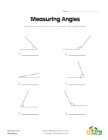## Measuring Angles Worksheet 1

Measure each of the angles with a protractor and w...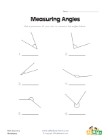## Measuring Angles Worksheet 2

Use a protractor to measure each of the angles.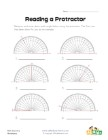## Reading a Protractor Worksheet 2

Read each protractor and write down the angles the...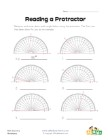## Reading a Protractor Worksheet 1

Read each protractor and write down the angles the...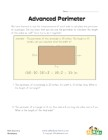## Advanced Perimeter Worksheet

Learn to use the perimeter to calculate the length...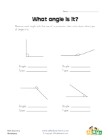## What Angle Is It?

Measure each angle and decide what type of angle i...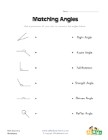## Matching Angles Worksheet

Draw a line to match each of the angles with the t...